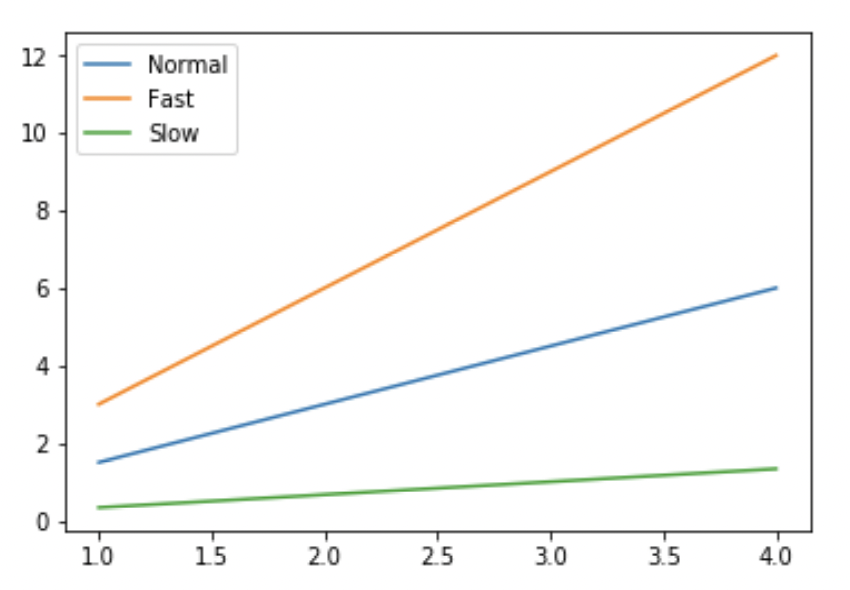# [Solved] Write a code to plot the speed of a passenger train as shown in the figure given below: Assume the values for x from 1 to 5 and the other axis is taken as 1.5x and x/3.0.

1
Topic starter

Write a code to plot the speed of a passenger train as shown in the figure given below: Assume the values for x from 1 to 5 and the other axis is taken as 1.5x and x/3.0.This topic was modified 10 months ago by CodeWithBishal-admin
Topic Tags
1
Topic starter
```import pandas as pd
import matplotlib.pyplot as plt
import numpy as np

x = np.arange(1, 5)

plt.plot(x, x*1.5, label='Normal')

plt.plot(x, x*3.0, label='Fast')

plt.plot(x, x/3.0, label='Slow')

plt.legend()

plt.show()```
This post was modified 10 months ago by CodeWithBishal-admin